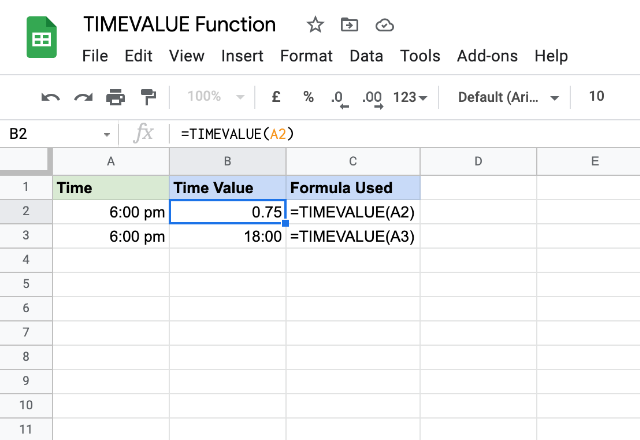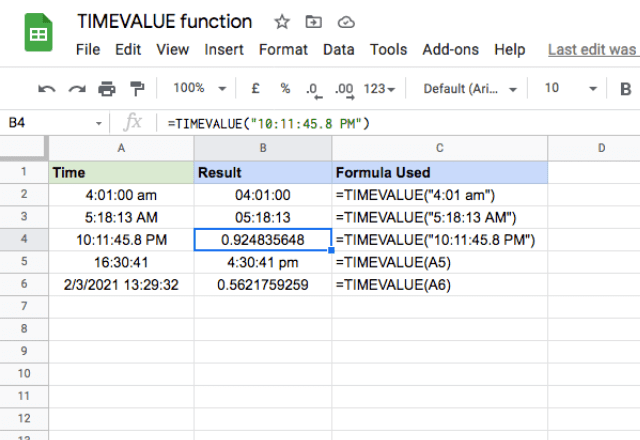# How to use the Google Sheets TIMEVALUE formulaWhen dealing with data in spreadsheets, having dates and times in string format is commonplace. While they convey the time, they may not be ideal for any kind of calculations or data processing. A way to get around that is to convert them into numerical time format. That is purpose of the TIMEVALUE formula in Google Sheets.

### Syntax

=TIMEVALUE(time_string)
• time_string – is the text string that represents time in either 12-hour or 24-hour time format. Like any other formulas that take in string parameters, you should enclose the time string in double quotes. You can also use a reference to the cell that holds the time string.

The TIMEVALUE function essentially converts a time string into a number format by expressing it as a fraction of a day. This fraction is in decimal terms, so for instance, 12:00 noon would be 0.5, since it’s halfway through the 24 hour day. The output of the TIMEVALUE function is therefore a number between 0 and 1.

To illustrate this further, see the example below. I have the time string of 6 pm, and I’m converting it to number format.In the first example here, I get the decimal value that I mentioned above. Because 6pm is ¾ of the way through a day, it is expressed in decimal form as 0.75. In the second example, I use the exact same formula but change the format of the cell using the Google Sheets toolbar. This changes the format from decimal form into a typical time form. The underlying number is still 0.75, but it’s expressed as 18:00:00, which is a bit easier on the eyes!

I’ll show a few more examples below. The first three examples accepted direct time strings (by typing them into the formula with quotation marks) and accordingly converted them into time values. The last two examples demonstrate the TIMEVALUE formula’s capability to accept cell references that have the time or date-time text strings. The time strings can be any of the following:

• hh AM/PM (12-hour format)
• hh:mm:ss (24-hour format)
• hh:mm:ss AM/PM (12-hour format)
• hh:mm (24-hour format)
• hh:mm AM/PM (12-hour format)There’s another interesting thing to note in the final example: the TIMEVALUE function can accept times with dates in the same cell. It simply ignores the date, and extracts the time. That can be extremely useful if you want to separate dates and times in your spreadsheet!

The TIMEVALUE function is also very precise. Looking at the last example, you can see how precise the decimal number is. You can also enter times down to a fraction of a second – in the example in row 4, I specify the seconds as 46.8 seconds!

You can leave the output of the TIMEVALUE function in number format, or convert it to any one of the available time formats in Google Sheets to suit your preference.

Editor’s note: This is a revised version of a previous post that has been updated for accuracy and comprehensiveness.